1.1bogo排序

1.2位运算

1.3打擂台

1.4morris遍历

2.1睡眠排序

2.2会死的兔子

2.3矩阵快速幂

2.4摔手机/摔鸡蛋

3.1斐波那契之美

3.2桶排序

3.3快速排序

3.4BFPRT

4.1防止新手错误的神级代码

4.2不用额外空间交换两个变量

4.3八皇后问题神操作

4.4马拉车——字符串神级算法

# 第一节

### 1.1bogo排序

Bogo排序(Bogo-sort)，又被称为猴子排序，是一种恶搞排序算法。

Bogo排序的最坏时间复杂度为O(∞)，一辈子也不能输出排序结果，平均时间复杂度为O(n·n!)。

public class Main {

public static void main(String[] args) {
int[] arr = { 9,8,7,6,5,4,3,2,1};
System.out.println("排序次数" + bogo(arr));
for (int i : arr) {
System.out.print(i + " ");
}
}

public static int bogo(int[] arr) {
int count = 0;
while (!isOrdered(arr)) {
shuffle(arr);
count++;
}
return count;
}

// 判断是否有序方法
public static boolean isOrdered(int[] arr) {
for (int i = 1; i < arr.length; i++) {
if (arr[i - 1] > arr[i]) {
return false;
}
}
return true;
}

// 随机排序方法
public static void shuffle(int[] arr) {
int temp;
for (int i = 0; i < arr.length; i++) {
int j = (int) (Math.random() * arr.length);
temp = arr[i];
arr[i] = arr[j];
arr[j] = temp;
}
}

}


9是中国最大的数字嘛，我就把数组长度设为9，结果平均排序次数要60万次，不知道我的运气怎么样哈哈，你们也试试吧？

### 1.2位运算

class Solution {
public int singleNumber(int[] nums) {
int ans = 0;
for(int i :nums)ans ^= i;
return ans;
}
}

### 1.3打擂台

class Solution {
public int majorityElement(int[] nums) {
int count = 0;//当前答案出现的次数
Integer candidate = null;//答案

for (int num : nums) {
if (count == 0) candidate = num;
count += (num == candidate) ? 1 : -1;
}

return candidate;
}
}

### 1.4morris遍历

void PreorderTraversal( BinTree BT )
{
if(BT==NULL)return ;
printf(" %c", BT->Data);
PreorderTraversal(BT->Left);
PreorderTraversal(BT->Right);
}

*p=root;
while(p || !st.empty())
{
if(p)//非空
{
//visit(p);进行操作
st.push(p);//入栈
p = p->lchild;左
}
else//空
{
p = st.top();//取出
st.pop();
p = p->rchild;//右
}
}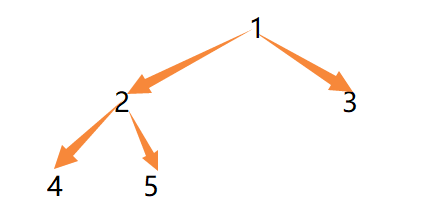1、如果没有左孩子，继续遍历右子树，比如：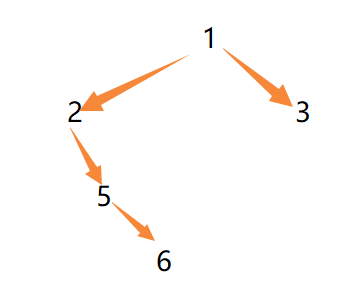2、如果有左孩子，找到左子树最右节点。

1）如果最右节点的右指针为空（说明第一次遇到），把它指向当前节点，当前节点向左继续处理。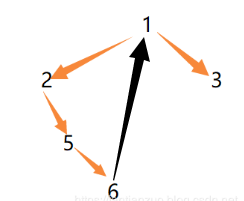2）如果最右节点的右指针不为空（说明它指向之前结点），把右指针设为空，当前节点向右继续处理。

	public static class Node {
public int value;
Node left;
Node right;

public Node(int data) {
this.value = data;
}
}

//打印时机（第一次遇到）：发现左子树最右的孩子右指针指向空，或无左子树。
public static void morrisPre(Node head) {
return;
}
Node cur2 = null;
while (cur1 != null) {
cur2 = cur1.left;
if (cur2 != null) {
while (cur2.right != null && cur2.right != cur1) {
cur2 = cur2.right;
}
if (cur2.right == null) {
cur2.right = cur1;
System.out.print(cur1.value + " ");
cur1 = cur1.left;
continue;
} else {
cur2.right = null;
}
} else {
System.out.print(cur1.value + " ");
}
cur1 = cur1.right;
}
System.out.println();
}

morris在发表文章时只写出了中序遍历。而先序遍历只是打印时机不同而已，所以后人改进出了先序遍历。至于后序，是通过打印所有的右边界来实现的：对每个有边界逆序，打印，再逆序回去。注意要原地逆序，否则我们morris遍历的意义也就没有了。

public class MorrisTraversal {
public static void process(Node head) {
return;
}
// 1

// 2

// 3
}

public static class Node {
public int value;
Node left;
Node right;
public Node(int data) {
this.value = data;
}
}

//打印时机：向右走之前
public static void morrisIn(Node head) {
return;
}
Node cur2 = null;//最右
while (cur1 != null) {
cur2 = cur1.left;
//左孩子不为空
if (cur2 != null) {
while (cur2.right != null && cur2.right != cur1) {
cur2 = cur2.right;
}//找到最右
//右指针为空，指向cur1，cur1向左继续
if (cur2.right == null) {
cur2.right = cur1;
cur1 = cur1.left;
continue;
} else {
cur2.right = null;
}//右指针不为空，设为空
}
System.out.print(cur1.value + " ");
cur1 = cur1.right;
}
System.out.println();
}

//打印时机（第一次遇到）：发现左子树最右的孩子右指针指向空，或无左子树。
public static void morrisPre(Node head) {
return;
}
Node cur2 = null;
while (cur1 != null) {
cur2 = cur1.left;
if (cur2 != null) {
while (cur2.right != null && cur2.right != cur1) {
cur2 = cur2.right;
}
if (cur2.right == null) {
cur2.right = cur1;
System.out.print(cur1.value + " ");
cur1 = cur1.left;
continue;
} else {
cur2.right = null;
}
} else {
System.out.print(cur1.value + " ");
}
cur1 = cur1.right;
}
System.out.println();
}

//逆序打印所有右边界
public static void morrisPos(Node head) {
return;
}
Node cur2 = null;
while (cur1 != null) {
cur2 = cur1.left;
if (cur2 != null) {
while (cur2.right != null && cur2.right != cur1) {
cur2 = cur2.right;
}
if (cur2.right == null) {
cur2.right = cur1;
cur1 = cur1.left;
continue;
} else {
cur2.right = null;
printEdge(cur1.left);
}
}
cur1 = cur1.right;
}
System.out.println();
}
//逆序打印
public static void printEdge(Node head) {
Node cur = tail;
while (cur != null) {
System.out.print(cur.value + " ");
cur = cur.right;
}
reverseEdge(tail);
}
//逆序（类似链表逆序）
public static Node reverseEdge(Node from) {
Node pre = null;
Node next = null;
while (from != null) {
next = from.right;
from.right = pre;
pre = from;
from = next;
}
return pre;
}
public static void main(String[] args) {

}

}

# 第二节

### 2.1睡眠排序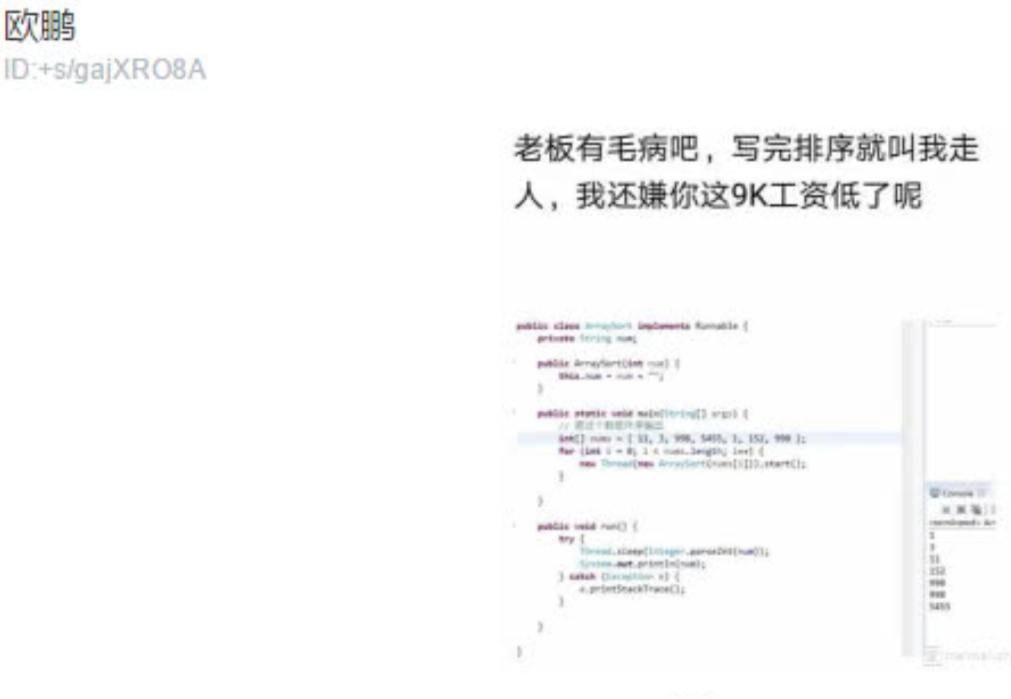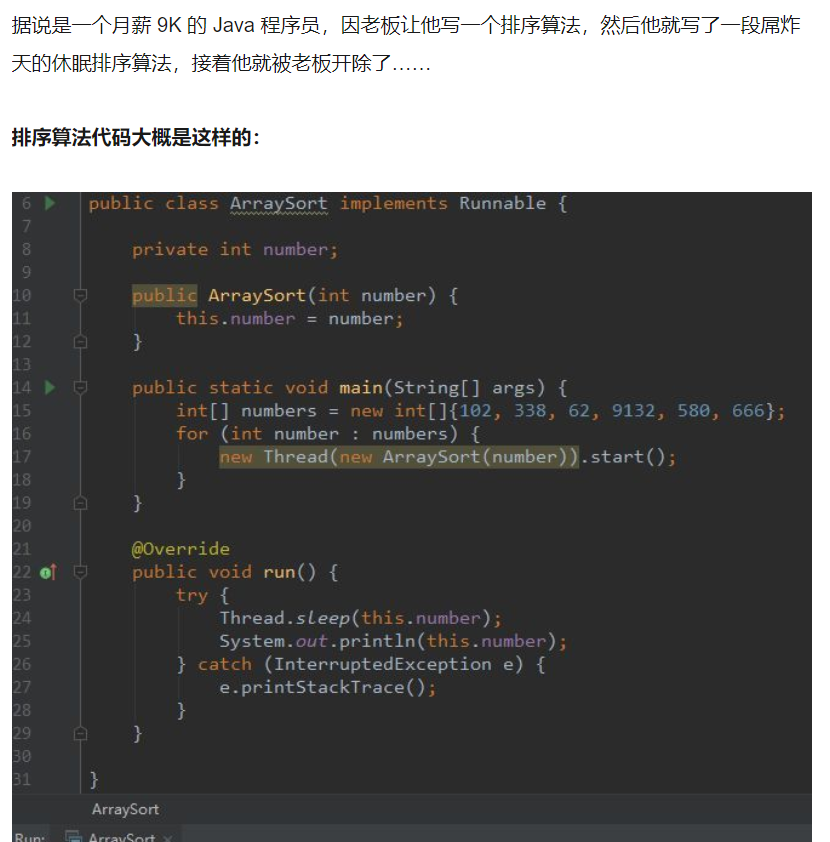### 2.2会死的兔子

F(n)=F(n-1)+F(n-2)。

def f(n):
if n==1 or n==2:
return 1
return f(n-1)+f(n-2)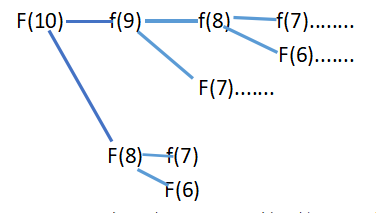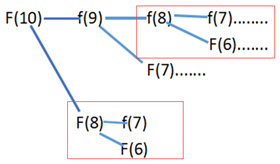def f1(n):
if n==1 or n==2:
return 1
l=*n                #保存结果
l,l=1,1            #赋初值
for i in range(2,n):
l[i]=l[i-1]+l[i-2]     #直接利用之前结果
return l[-1]

def f2(n):
a,b=1,1
for i in range(n-1):
a,b=b,a+b
return a

### 2.3矩阵快速幂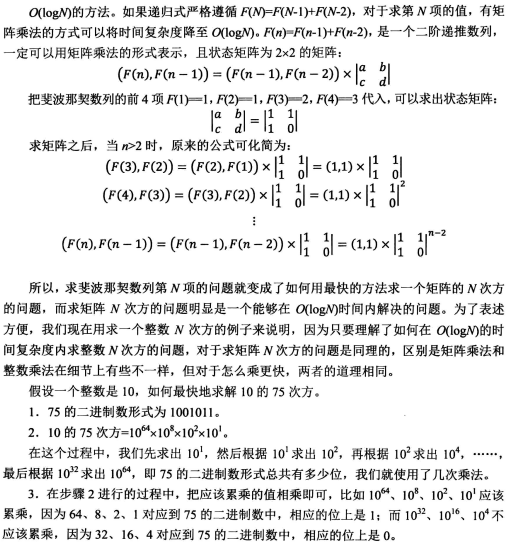public static int[][] matrixPower(int[][] m, int p) {
int[][] res = new int[m.length][m.length];
for (int i = 0; i < res.length; i++) {
res[i][i] = 1;
}
int[][] tmp = m;
for (; p != 0; p >>= 1) {
if ((p & 1) != 0) {
res = muliMatrix(res, tmp);
}
tmp = muliMatrix(tmp, tmp);
}
return res;
}

public static int[][] muliMatrix(int[][] m1, int[][] m2) {
int[][] res = new int[m1.length][m2.length];
for (int i = 0; i < m1.length; i++) {
for (int j = 0; j < m2.length; j++) {
for (int k = 0; k < m2.length; k++) {
res[i][j] += m1[i][k] * m2[k][j];
}
}
}
return res;
}
	public static int f3(int n) {
if (n < 1) {
return 0;
}
if (n == 1 || n == 2) {
return 1;
}
int[][] base = { { 1, 1 }, { 1, 0 } };
int[][] res = matrixPower(base, n - 2);
return res + res;
}

### 2.4摔手机/摔鸡蛋

leetcode的hard难度（我认为应该出一个顶级难度）：

x星球的居民脾气不太好，但好在他们生气的时候唯一的异常举动是：摔手机。

x星球有很多高耸入云的高塔，刚好可以用来做耐摔测试。塔的每一层高度都是一样的，与地球上稍有不同的是，他们的第一层不是地面，而是相当于我们的2楼。
如果手机从第7层扔下去没摔坏，但第8层摔坏了，则手机耐摔指数=7。

为了减少测试次数，从每个厂家抽样3部手机参加测试。
某次测试的塔高为1000层，如果我们总是采用最佳策略，在最坏的运气下最多需要测试多少次才能确定手机的耐摔指数呢？
请填写这个最多测试次数。

注意：需要填写的是一个整数，不要填写任何多余内容

### 时空复杂度目录

DP:                            O(N*N*K)  O(N*K)

### 尝试较优的策略

（以上几行是为了让读者理解决策，下面正文分析）

### 归纳表达式

f（n，k）=min（  max（f(i-1,k-1) ，f(n-i,k) ） i取1-n任意数    ）+1

### 写出暴力递归

	public static int solution1(int nLevel, int kChess) {
if (nLevel == 0) {
return 0;
}//范围缩小至0
if (kChess == 1) {
return nLevel;
}//每层依次试
int min = Integer.MAX_VALUE;//取不影响结果的数
for (int i = 1; i != nLevel + 1; i++) {
//尝试所有决策，取最优
min = Math.min(
min,
Math.max(Process1(i - 1, kChess - 1),Process1(nLevel - i, kChess)));
}
return min + 1;//别忘了加上本次
}

### 改为动态规划

https://blog.csdn.net/hebtu666/article/details/79912328

	public static int solution2(int nLevel, int kChess) {
if (kChess == 1) {
return nLevel;
}
int[][] dp = new int[nLevel + 1][kChess + 1];
for (int i = 1; i != dp.length; i++) {
dp[i] = i;
}
for (int i = 1; i != dp.length; i++) {
for (int j = 2; j != dp.length; j++) {
int min = Integer.MAX_VALUE;
for (int k = 1; k != i + 1; k++) {
min = Math.min(min,
Math.max(dp[k - 1][j - 1], dp[i - k][j]));
}
dp[i][j] = min + 1;
}
}
return dp[nLevel][kChess];
}

### 压缩空间

	public static int solution3(int nLevel, int kChess) {
if (kChess == 1) {
return nLevel;
}
int[] preArr = new int[nLevel + 1];
int[] curArr = new int[nLevel + 1];
for (int i = 1; i != curArr.length; i++) {
curArr[i] = i;
}//初始化
for (int i = 1; i != kChess; i++) {
//先交换
int[] tmp = preArr;
preArr = curArr;
curArr = tmp;
//然后打新的一行
for (int j = 1; j != curArr.length; j++) {
int min = Integer.MAX_VALUE;
for (int k = 1; k != j + 1; k++) {
min = Math.min(min, Math.max(preArr[k - 1], curArr[j - k]));
}
curArr[j] = min + 1;
}
}
return curArr[curArr.length - 1];
}

### 四边形不等式优化

(mk[i+1,j]-md[i+1,j])-(mk[i,j]-md[i,j])

=(mk[i+1,j]+md[i,j])-(md[i+1,j]+mk[i,j])

=(m[i+1,k]+m[k,j]+m[i,d]+m[d,j])-(m[i+1,d]+m[d,j]+m[i,k]+m[k,j])

=(m[i+1,k]+m[i,d])-(m[i+1,d]+m[i,k])

∵m满足四边形不等式，∴对于i<i+1≤k<d有m[i+1,k]+m[i,d]≥m[i+1,d]+m[i,k]

∴(mk[i+1,j]-md[i+1,j])≥(mk[i,j]-md[i,j])≥0

∴s[i,j]≤s[i+1,j]，同理可证s[i,j-1]≤s[i,j]

	public static int solution4(int nLevel, int kChess) {
if (kChess == 1) {
return nLevel;
}
int[][] dp = new int[nLevel + 1][kChess + 1];
for (int i = 1; i != dp.length; i++) {
dp[i] = i;
}
int[] cands = new int[kChess + 1];
for (int i = 1; i != dp.length; i++) {
dp[i] = 1;
cands[i] = 1;
}
for (int i = 2; i < nLevel + 1; i++) {
for (int j = kChess; j > 1; j--) {
int min = Integer.MAX_VALUE;
int minEnum = cands[j];
int maxEnum = j == kChess ? i / 2 + 1 : cands[j + 1];
//优化策略
for (int k = minEnum; k < maxEnum + 1; k++) {
int cur = Math.max(dp[k - 1][j - 1], dp[i - k][j]);
if (cur <= min) {
min = cur;
cands[j] = k;//最优解记录层数
}
}
dp[i][j] = min + 1;
}
}
return dp[nLevel][kChess];
}

### 换一种思路

（比如训练时一道贪心我死活往dp想，肝了两个小时以后，不主攻这个方向的队友三分钟就有贪心思路了，泪目，不要把简单问题复杂化

### 最优解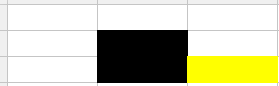public static int solution5(int nLevel, int kChess) {
int bsTimes = log2N(nLevel) + 1;
if (kChess >= bsTimes) {
return bsTimes;
}
int[] dp = new int[kChess];
int res = 0;
while (true) {
res++;//压缩空间记得记录次数
int previous = 0;
for (int i = 0; i < dp.length; i++) {
int tmp = dp[i];
dp[i] = dp[i] + previous + 1;
previous = tmp;
if (dp[i] >= nLevel) {
return res;
}
}
}
}

public static int log2N(int n) {
int res = -1;
while (n != 0) {
res++;
n >>>= 1;
}
return res;
}

### 测试：

DP:                            O(N*N*K)  O(N*K)

		long start = System.currentTimeMillis();
solution1(30, 2);
long end = System.currentTimeMillis();
System.out.println("cost time: " + (end - start) + " ms");
start = System.currentTimeMillis();
solution2(30, 2);
end = System.currentTimeMillis();
System.out.println("cost time: " + (end - start) + " ms");
start = System.currentTimeMillis();
solution3(30, 2);
end = System.currentTimeMillis();
System.out.println("cost time: " + (end - start) + " ms");
start = System.currentTimeMillis();
solution4(30, 2);
end = System.currentTimeMillis();
System.out.println("cost time: " + (end - start) + " ms");
start = System.currentTimeMillis();
solution5(30, 2);
end = System.currentTimeMillis();
System.out.println("cost time: " + (end - start) + " ms");
/*

cost time: 7043 ms
cost time: 0 ms
cost time: 0 ms
cost time: 0 ms
cost time: 0 ms
*/

solution(100000, 10):

solution2 cost time: 202525 ms
solution3 cost time: 38131 ms
solution4 cost time: 11295 ms
solution5 cost time: 0 ms

solution5(1000 000 000,100):0 ms

solution5(1000 000 000,10):0 ms

# 第三节

### 3.1斐波那契之美

1. 随着数列项数的增加，前一项与后一项之比越来越逼近黄金分割的数值0.6180339887..
2. 从第二项开始，每个奇数项的平方都比前后两项之积多1，每个偶数项的平方都比前后两项之积少1。（注：奇数项和偶数项是指项数的奇偶，而并不是指数列的数字本身的奇偶，比如第五项的平方比前后两项之积多1，第四项的平方比前后两项之积少1）
3. 斐波那契数列的第n项同时也代表了集合{1,2,…,n}中所有不包含相邻正整数的子集个数。
4. f(0)+f(1)+f(2)+…+f(n)=f(n+2)-1
5. f(1)+f(3)+f(5)+…+f(2n-1)=f(2n)
6. f(2)+f(4)+f(6)+…+f(2n) =f(2n+1)-1
7. [f(0)]^2+[f(1)]^2+…+[f(n)]^2=f(n)·f(n+1)
8. f(0)-f(1)+f(2)-…+(-1)^n·f(n)=(-1)^n·[f(n+1)-f(n)]+1
9. f(m+n)=f(m-1)·f(n-1)+f(m)·f(n) (利用这一点，可以用程序编出时间复杂度仅为O（log n）的程序。)

### 3.2桶排序

l=list(map(int,input().split(" ")))
n=max(l)-min(l)
p=*(n+1)#为了省空间
for i in l:
p[i-min(l)]=1#去重排序，做标记即可
for i in range(n):
if p[i]==1:#判断是否出现过
print(i+min(l),end=" ")

### 3.3快速排序

”荷兰国旗难题“是计算机科学中的一个程序难题，它是由Edsger Dijkstra提出的。荷兰国旗是由红、白、蓝三色组成的。

3
BBRRWBWRRR
RRRWWRWRB
RBRW

RRRRRWWBBB
RRRRRWWWB
RRWB

(2)如果遍历到的位置为2，说明属于中部，now++；

(3)如果遍历到的位置为3，说明属于后部，于是就和tail交换值，然而，如果此时交换后now指向的值属于前部，那么就执行(1)，tail--；

#include<iostream>
#include<algorithm>
using namespace std;

const int maxn = 100 + 5;

int n;
string str;
int main(){
cin>>n;
while(n--){
cin>>str;
int len=str.size();
int now=0,ans=0;
while(now<=tail){
if(str[now]=='R'){
now++;
}
else if(str[now]=='W'){
now++;
}
else{
swap(str[now],str[tail]);
tail--;
}
}
cout<<str<<endl;
}return 0;
}

def gg(a,b):
global l
if a>=b:#注意停止条件，我以前没加>卡了半小时
return
x,y=a,b
import random#为了避免遇到基本有序序列退化，随机选点
g=random.randint(a,b)
l[g],l[y]=l[y],l[g]#交换选中元素和末尾元素
while a<b:
if l[a]>l[y]:#比目标元素大
l[a],l[b-1]=l[b-1],l[a]#交换
b=b-1#大于区扩大
#注意：换过以后a不要加，因为新换过来的元素并没有判断过
else:a=a+1#小于区扩大
l[y],l[a]=l[a],l[y]#这时a=b
#现在解释a和b：a的意义是小于区下一个元素
#b是大于区的第一个元素
gg(x,a-1)#左右分别递归
gg(a+1,y)

l=list(map(int,input().split(" ")))
gg(0,len(l)-1)
print(l)

### 3.4BFPRT

1、将n个元素划分为n/5个组，每组5个元素
2、对每组排序，找到n/5个组中每一组的中位数；
3、对于找到的所有中位数，调用BFPRT算法求出它们的中位数，作为划分值。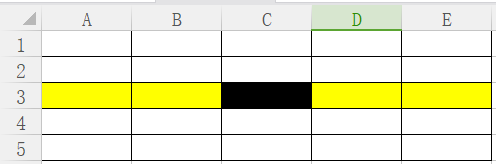public class BFPRT {
//前k小
public static int[] getMinKNumsByBFPRT(int[] arr, int k) {
if (k < 1 || k > arr.length) {
return arr;
}
int minKth = getMinKthByBFPRT(arr, k);
int[] res = new int[k];
int index = 0;
for (int i = 0; i != arr.length; i++) {
if (arr[i] < minKth) {
res[index++] = arr[i];
}
}
for (; index != res.length; index++) {
res[index] = minKth;
}
return res;
}
//第k小
public static int getMinKthByBFPRT(int[] arr, int K) {
int[] copyArr = copyArray(arr);
return select(copyArr, 0, copyArr.length - 1, K - 1);
}

public static int[] copyArray(int[] arr) {
int[] res = new int[arr.length];
for (int i = 0; i != res.length; i++) {
res[i] = arr[i];
}
return res;
}
//给定一个数组和范围，求第i小的数
public static int select(int[] arr, int begin, int end, int i) {
if (begin == end) {
return arr[begin];
}
int pivot = medianOfMedians(arr, begin, end);//划分值
int[] pivotRange = partition(arr, begin, end, pivot);
if (i >= pivotRange && i <= pivotRange) {
return arr[i];
} else if (i < pivotRange) {
return select(arr, begin, pivotRange - 1, i);
} else {
return select(arr, pivotRange + 1, end, i);
}
}
//在begin end范围内进行操作
public static int medianOfMedians(int[] arr, int begin, int end) {
int num = end - begin + 1;
int offset = num % 5 == 0 ? 0 : 1;//最后一组的情况
int[] mArr = new int[num / 5 + offset];//中位数组成的数组
for (int i = 0; i < mArr.length; i++) {
int beginI = begin + i * 5;
int endI = beginI + 4;
mArr[i] = getMedian(arr, beginI, Math.min(end, endI));
}
return select(mArr, 0, mArr.length - 1, mArr.length / 2);
//只不过i等于长度一半，用来求中位数
}
//经典partition过程
public static int[] partition(int[] arr, int begin, int end, int pivotValue) {
int small = begin - 1;
int cur = begin;
int big = end + 1;
while (cur != big) {
if (arr[cur] < pivotValue) {
swap(arr, ++small, cur++);
} else if (arr[cur] > pivotValue) {
swap(arr, cur, --big);
} else {
cur++;
}
}
int[] range = new int;
range = small + 1;
range = big - 1;
return range;
}
//五个数排序，返回中位数
public static int getMedian(int[] arr, int begin, int end) {
insertionSort(arr, begin, end);
int sum = end + begin;
int mid = (sum / 2) + (sum % 2);
return arr[mid];
}
//手写排序
public static void insertionSort(int[] arr, int begin, int end) {
for (int i = begin + 1; i != end + 1; i++) {
for (int j = i; j != begin; j--) {
if (arr[j - 1] > arr[j]) {
swap(arr, j - 1, j);
} else {
break;
}
}
}
}
//交换值
public static void swap(int[] arr, int index1, int index2) {
int tmp = arr[index1];
arr[index1] = arr[index2];
arr[index2] = tmp;
}
//打印
public static void printArray(int[] arr) {
for (int i = 0; i != arr.length; i++) {
System.out.print(arr[i] + " ");
}
System.out.println();
}

public static void main(String[] args) {
int[] arr = { 6, 9, 1, 3, 1, 2, 2, 5, 6, 1, 3, 5, 9, 7, 2, 5, 6, 1, 9 };
// sorted : { 1, 1, 1, 1, 2, 2, 2, 3, 3, 5, 5, 5, 6, 6, 6, 7, 9, 9, 9 }
printArray(getMinKNumsByBFPRT(arr, 10));

}
}

# 第四节

### 4.1防止新手错误的神级代码

#define ture true

#define flase false

#difine viod void

#define mian main

#define ； ;

### 4.2不用额外空间交换两个变量

a = 5
b = 8

#计算a和b两个点到原点的距离之和，并且赋值给a
a = a+b

#使用距离之和减去b到原点的距离
#a-b 其实就是a的原值（a到原点的距离），现在赋值给了b
b = a-b

#再使用距离之和减去b (a到原点的距离)
#得到的是b的原值（b到原点的距离），现在赋值给了a
a = a-b

### 4.3八皇后问题神操作

（本文不展开讲状态压缩，以后再说）

N*N的二维数组，在每一个位置进行尝试，在当前位置上判断是否满足放置皇后的条件（这一点的行、列、对角线上，没有皇后）。

public class NQueens {
public static int num1(int n) {
if (n < 1) {
return 0;
}
int[] record = new int[n];
return process1(0, record, n);
}
public static int process1(int i, int[] record, int n) {
if (i == n) {
return 1;
}
int res = 0;
for (int j = 0; j < n; j++) {
if (isValid(record, i, j)) {
record[i] = j;
res += process1(i + 1, record, n);
}
}//对于当前行，依次尝试每列
return res;
}
//判断当前位置是否可以放置
public static boolean isValid(int[] record, int i, int j) {
for (int k = 0; k < i; k++) {
if (j == record[k] || Math.abs(record[k] - j) == Math.abs(i - k)) {
return false;
}
}
return true;
}
public static void main(String[] args) {
int n = 8;
System.out.println(num1(n));
}
}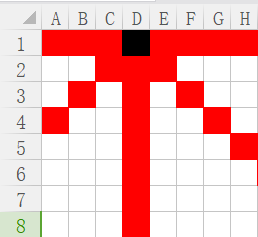1）本行影响不提，根据优化一已经避免

2）本列影响，一直影响D列，直到第一行在D放棋子的所有情况结束。

3）左斜线：每向下一行，实际上对当前行的影响区域就向左移动

4）右斜线则相反：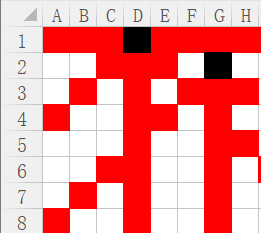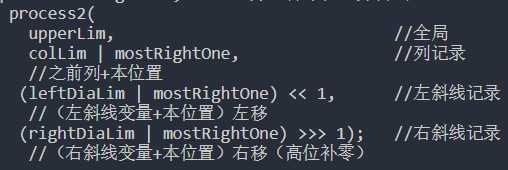public class NQueens {
public static int num2(int n) {
// 因为本方法中位运算的载体是int型变量，所以该方法只能算1~32皇后问题
// 如果想计算更多的皇后问题，需使用包含更多位的变量
if (n < 1 || n > 32) {
return 0;
}
int upperLim = n == 32 ? -1 : (1 << n) - 1;
//upperLim的作用为棋盘大小，比如8皇后为00000000 00000000 00000000 11111111
//32皇后为11111111 11111111 11111111 11111111
return process2(upperLim, 0, 0, 0);
}

public static int process2(int upperLim, int colLim, int leftDiaLim,
int rightDiaLim) {
if (colLim == upperLim) {
return 1;
}
int pos = 0;            //pos：所有的合法位置
int mostRightOne = 0;   //所有合法位置的最右位置

//所有记录按位或之后取反，并与全1按位与，得出所有合法位置
pos = upperLim & (~(colLim | leftDiaLim | rightDiaLim));
int res = 0;//计数
while (pos != 0) {
mostRightOne = pos & (~pos + 1);//取最右的合法位置
pos = pos - mostRightOne;       //去掉本位置并尝试
res += process2(
upperLim,                             //全局
colLim | mostRightOne,                //列记录
//之前列+本位置
(leftDiaLim | mostRightOne) << 1,      //左斜线记录
//（左斜线变量+本位置）左移
(rightDiaLim | mostRightOne) >>> 1);   //右斜线记录
//（右斜线变量+本位置）右移（高位补零）
}
return res;
}

public static void main(String[] args) {
int n = 8;
System.out.println(num2(n));
}
}


32皇后：结果/时间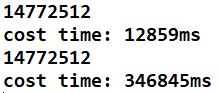public class NQueens {

public static int num1(int n) {
if (n < 1) {
return 0;
}
int[] record = new int[n];
return process1(0, record, n);
}

public static int process1(int i, int[] record, int n) {
if (i == n) {
return 1;
}
int res = 0;
for (int j = 0; j < n; j++) {
if (isValid(record, i, j)) {
record[i] = j;
res += process1(i + 1, record, n);
}
}
return res;
}

public static boolean isValid(int[] record, int i, int j) {
for (int k = 0; k < i; k++) {
if (j == record[k] || Math.abs(record[k] - j) == Math.abs(i - k)) {
return false;
}
}
return true;
}

public static int num2(int n) {
if (n < 1 || n > 32) {
return 0;
}
int upperLim = n == 32 ? -1 : (1 << n) - 1;
return process2(upperLim, 0, 0, 0);
}

public static int process2(int upperLim, int colLim, int leftDiaLim,
int rightDiaLim) {
if (colLim == upperLim) {
return 1;
}
int pos = 0;
int mostRightOne = 0;
pos = upperLim & (~(colLim | leftDiaLim | rightDiaLim));
int res = 0;
while (pos != 0) {
mostRightOne = pos & (~pos + 1);
pos = pos - mostRightOne;
res += process2(upperLim, colLim | mostRightOne,
(leftDiaLim | mostRightOne) << 1,
(rightDiaLim | mostRightOne) >>> 1);
}
return res;
}

public static void main(String[] args) {
int n = 32;

long start = System.currentTimeMillis();
System.out.println(num2(n));
long end = System.currentTimeMillis();
System.out.println("cost time: " + (end - start) + "ms");

start = System.currentTimeMillis();
System.out.println(num1(n));
end = System.currentTimeMillis();
System.out.println("cost time: " + (end - start) + "ms");
}
}


### 4.4马拉车——字符串神级算法

Manacher's Algorithm 马拉车算法操作及原理

package advanced_001;

public class Code_Manacher {

public static char[] manacherString(String str) {
char[] charArr = str.toCharArray();
char[] res = new char[str.length() * 2 + 1];
int index = 0;
for (int i = 0; i != res.length; i++) {
res[i] = (i & 1) == 0 ? '#' : charArr[index++];
}
return res;
}

public static int maxLcpsLength(String str) {
if (str == null || str.length() == 0) {
return 0;
}
char[] charArr = manacherString(str);
int[] pArr = new int[charArr.length];
int C = -1;
int R = -1;
int max = Integer.MIN_VALUE;
for (int i = 0; i != charArr.length; i++) {
pArr[i] = R > i ? Math.min(pArr[2 * C - i], R - i) : 1;
while (i + pArr[i] < charArr.length && i - pArr[i] > -1) {
if (charArr[i + pArr[i]] == charArr[i - pArr[i]])
pArr[i]++;
else {
break;
}
}
if (i + pArr[i] > R) {
R = i + pArr[i];
C = i;
}
max = Math.max(max, pArr[i]);
}
return max - 1;
}

public static void main(String[] args) {
String str1 = "abc1234321ab";
System.out.println(maxLcpsLength(str1));
}

}


问题：查找一个字符串的  最长回文子串

当然，不一样。子序列可以不连续，子串必须连续。

子序列，每个元素都可以选或者不选，所以有2的n次方个子序列（包括空）

(这里有一个思想需要注意，遇到等差数列求和，基本都是o(n^2)级别的)

### 4.4二、初步优化

0位置，左边没东西，只有自己；

1位置，判断左边右边是否相等，1=1所以接着扩，然后左边没了，所以以1位置为对称轴的最长回文长度就是3；

2位置，左右都是2，相等，继续，左右都是1，继续，左边没了，所以最长为5

3位置，左右开始扩，1=1，2=2，1=1，左边没了，所以长度是7

### 假设遍历到位置i，如何操作呢1）i>R:也就是说，i以及i右边，我们根本不知道是什么，因为从来没扩到那里。那没有任何优化，直接往右暴力 扩呗。

（下面我们做i关于c的对称点，i’）

2）i<R:，

i’的回文左边界在c回文左边界的里面

i’回文左边界在整体回文的外面

i’左边界和c左边界是一个元素

(怕你忘了概念，c是对称中心，c它当初扩到了R，R是目前扩到的最右的地方，现在咱们想以i为中心，看能扩到哪里。)

2-1）i’的回文左边界在c回文的里面：看图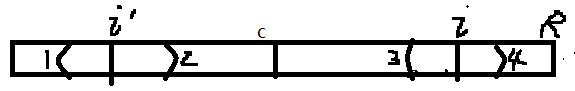由此得出结论1：

2-2）i回文左边界在整体回文的外面了：看图这时，我们也可以直接确定i能扩到哪里，请听分析：

2’为2关于i’的对称点，当初i’左右为什么能继续扩呢？说明点2=点2’---------结论2；

2-3）最后一种当然是i左边界和c左边界是一个元素点1！=点2，点2=点3，就只能推出这些，只知道34中间肯定是回文，外边的呢？不知道啊，因为不知道3和4相不相等，所以我们得出结论：点3点4内肯定是，继续暴力扩。

### 4.4四、代码及复杂度分析

看代码大家是不是觉得不像o(n)？其实确实是的，来分析一波。。

（要是写四种情况当然也可以。。但是我懒的写，太多了。便于理解分了四种情况解释，code整合后就是这样子）

12-183万+01-112596
06-192189
12-085万+
05-145568
03-291525
03-031万+
06-08229
06-12105
01-11158